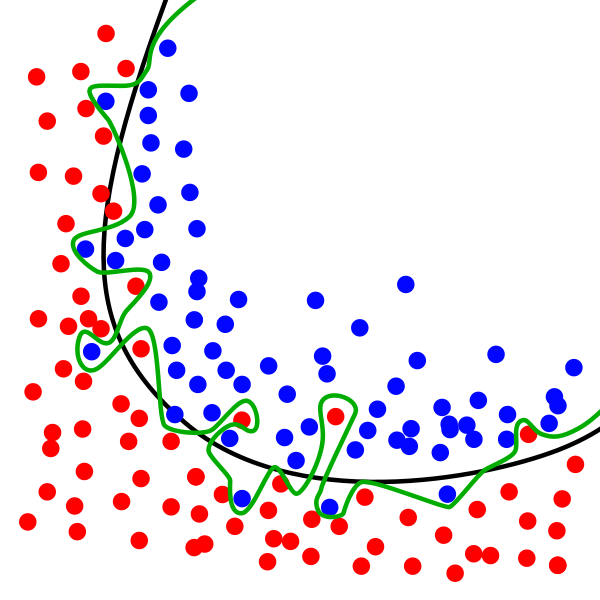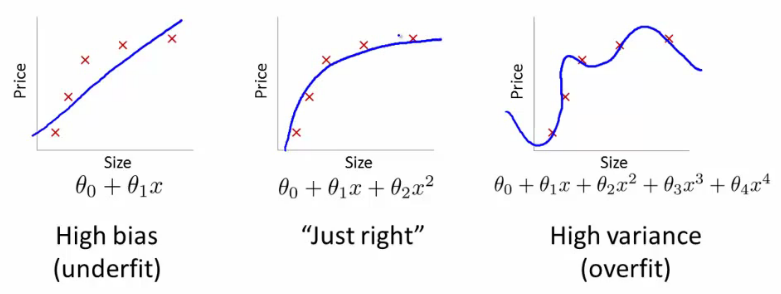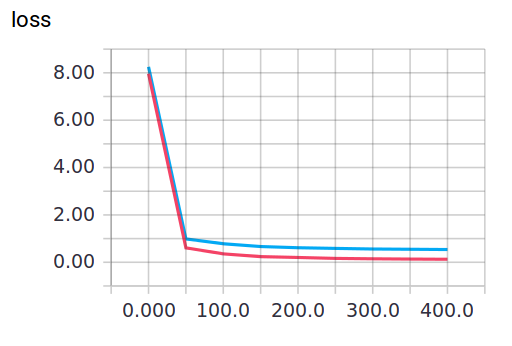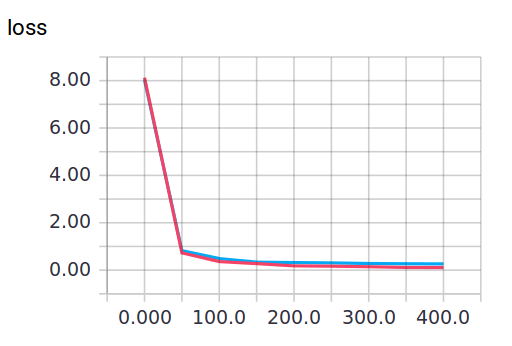# Dropout 解决 overfitting

## 要定 ¶

Overfitting 也被称为过度学习，过度拟合。 它是机器学习中常见的问题。 举个Classification（分类）的例子。## 建立 dropout 层 ¶

import tensorflow as tf
from sklearn.datasets import load_digits
from sklearn.cross_validation import train_test_split
from sklearn.preprocessing import LabelBinarizer

keep_prob = tf.placeholder(tf.float32)
...
...
Wx_plus_b = tf.nn.dropout(Wx_plus_b, keep_prob)


digits = load_digits()
X = digits.data
y = digits.target
y = LabelBinarizer().fit_transform(y)
X_train, X_test, y_train, y_test = train_test_split(X, y, test_size=.3)


# add output layer
l1 = add_layer(xs, 64, 50, 'l1', activation_function=tf.nn.tanh)
prediction = add_layer(l1, 50, 10, 'l2', activation_function=tf.nn.softmax)


loss函数（即最优化目标函数）选用交叉熵函数。交叉熵用来衡量预测值和真实值的相似程度，如果完全相同，交叉熵就等于零。

cross_entropy = tf.reduce_mean(-tf.reduce_sum(ys * tf.log(prediction),
reduction_indices=))  # loss


train方法（最优化算法）采用梯度下降法。

train_step = tf.train.GradientDescentOptimizer(0.5).minimize(cross_entropy)


## 训练 ¶

sess.run(train_step, feed_dict={xs: X_train, ys: y_train, keep_prob: 0.5})
#sess.run(train_step, feed_dict={xs: X_train, ys: y_train, keep_prob: 1})


## 可视化结果 ¶

keep_prob=1时，模型对训练数据的适应性优于测试数据，存在overfitting，输出如下： 红线是 train 的误差, 蓝线是 test 的误差.keep_prob=0.5时效果好了很多，输出如下：